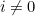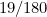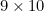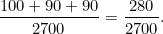# An almighty coincidence

December 2007

First let's calculate the frequency of a particular digitwith.

Its frequency among 1-digit numbers is clearly 1/9.

Within the 90 two-digit numbers our digitcan occur in first or in second place. If it occurs in first place, it can be followed by any of the digits from 0 to 9, so there are 10 instances of this. If it occurs in second place it can be preceded by the digits from 1 up to 9, so there are 9 instances of this. Now if we write out all 90 two-digit numbers, we get a string of 180 digits in total, so the frequency ofin this string is.

Within the 900 three-digit numbers the digitcan occur in first, second or third place. If it appears in first place, it can be followed by any of the numbers from 0 to 99, so there are 100 instances of this. If it occurs in second place, it can be preceded by any of the numbers from 1 to 9, and followed by any of the numbers from 0 to 9, so there areinstances ofoccurring in the middle. Ifoccurs in last place, it can be preceded by any of the numbers from 10 to 99, so there are 90 instances of this.

If we write out all the 900 three-digit numbers, we get a string of 2700 digits in total, so the frequency ofamong 3-digit numbers isThe overall result now follows from the fact that 1, 2 and 3-digit numbers make up, respectively, 1/999th, 90/999th and 900/999th of all the numbers from 1 to 999.

A similar calculation gives the result for the digit 0.